# Time Card/Sheet with overtime Calculator

With this overtime timecard calculator, you can make a daily or weekly timesheet. For the weekly timesheet, enter the check-in and check-out timing, total them to find the daily overtime, and then grand total them to see the weekly overtime done. The AM and PM format makes it easy to add the time. This sheet can be emailed to the recipient by entering the email address and can also be printed.

1. Enter Time in 24 hour Formate.
2. You can Enter the timings from Monday to Sunday.
3. Use IN Box to Enter the time of work starting.
4. Use OUT Box to Enter time of leaving.
5. There are three Shifts for one day. Use according to your requirements.
6. Get Results of each day total Hours in the Pink Box below each day.
7. Enter the Date OF pay Slip.
8. Enter Over time if any.
9. Enter Employe Name In Name Box(Yellow button).
10. Enter Hourly Rate\$ in box against \$ per Hour Button.
11. You will get Total Amount to be paid in Amount Box
12. Use Clear Button to Clean the entire sheet written.
13. Use Print Button To Print the complete TimeCard.
14. Use Email Box if you want to recieve the complete TimeCard at your Email ID.

(Please enter hours in 24 hour format)

{{parseInt(timeSlot.dayTime/60)}} Hr {{timeSlot.dayTime%60}} MinAbout the Author Nauman Rehmat Nauman is a Digital Marketing Specialist and owner of several online tools like DrEmployee. He believes in helping common people by providing a free online solution to day to day tasks. This project is one of them to offer free financial tools and tips.

## How to Use Time Card/Sheet with Overtime Calculator?

Want to know how productive you are? Or what is your actual worked hours without lunch breaks? Or want to see the duration of your several daily tasks? Then our Timesheet with Overtime Calculator is just for you. It allows you to calculate the length of 3 different tasks in a day and 21 various tasks in a week. Moreover, it can help you figure Total Hours Worked in a Day and a Week even if you have unpaid lunch breaks or if you work overtime. So without wasting any of your precious time, let's jump onto the step by step guide on how to use Timesheet with Overtime Calculator.

For better understanding, we will use an example of an employee who needs to calculate his total Pay for a week, where his lunch breaks are unpaid, but his site visits are paid, and he works overtime (paid after 40 hours in a week).

We have also divided the calculator into four sections, which are:

1. Section 1, which has 7 Tables with fields, each table for each day, having the option to calculate the Time Duration for 3 Tasks for each day.

Note: Click on the Tabs to navigate between the days. Also, your data entered won't be deleted during navigation.

1. Section 2 has a form that gives you the ability to enter your Name, Date, and displays the total duration of tasks in a week. This calculator is mostly used for Working Hours calculation, so this form will view total Hours worked in a week.
2. Section 3 lets you enter your hourly rate and to choose your overtime payment criteria and display your overtime hours (If any).
3. Section 4 displays total Pay for a Week according to your hourly rate and overtime.

Step 1: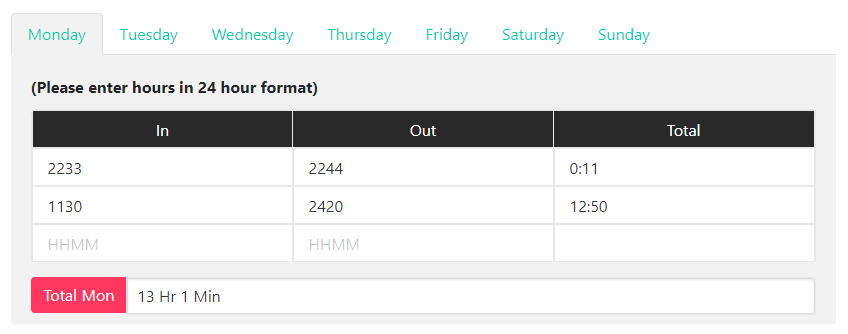1. Upon Launching the Timesheet with Overtime Calculator, you will see the section 1 of the calculator with an interface as shown in the image above.
2. So sticking on with our example, the employee will now enter his Time IN and Time OUT of work before lunch break on the first row of the table in 24 Hours Format (Military Time).

Note: You can also convert your regular Time into Military time using our Military Time Converter.

1. Next, He will Enter the Start Time and End Time of his second shift after the lunch break.
2. In the last field, he enters the Start Time and End Time of Site Visits that are paid.
3. Now, his total hours and minutes worked on Monday will appear next to the red "Total Mon" Label, as shown in the image above.

Step 2:

Now the employee will repeat Step 1 to fill all the 7 Tables in section 1, assigned to each day in a week to get total hours and minutes worked on each day in a week.

Step 3: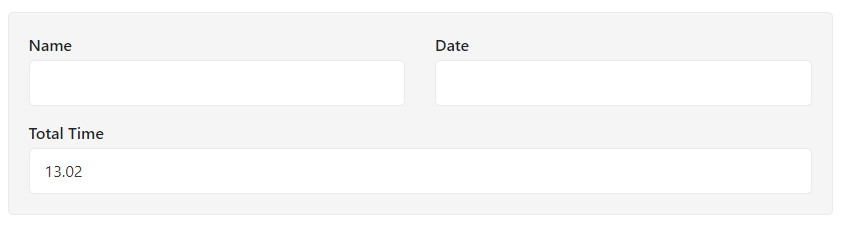1. After 7 Tables in section 1, comes Section 2 with an interface, as shown in the image above.
2. Here the employee needs to enter his Name and
3. He can also see his total hours and minutes worked in a Week on the "Total Time"

Step 4: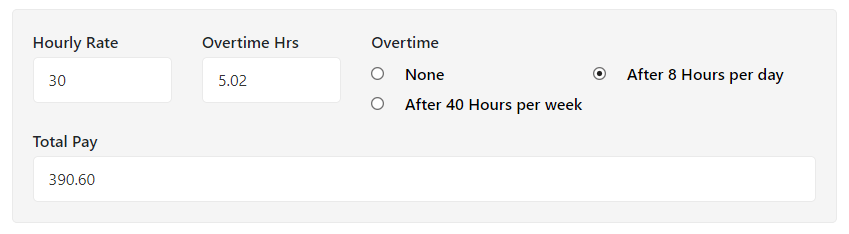1. Once the employee completes Section 2, He moves on to Section 3 that has an interface, as shown in the image above.
2. In the First field, He enters his Hourly rate.
3. In the second field, he chooses his overtime criteria, which as according to the example, is "After 40 Hours per Week," which means He would be paid overtime for every extra hour he works after his regular 40 hours in a week.
4. Upon choosing the overtime criteria, his overtime hours would automatically be displayed in the last field with the label "Overtime Hrs" according to the details he has entered in Section 1.
1. Once all of the details are entered in Section 1, 2, and 3, the Total Pay of an Employee will be displayed in the "Total Pay" Field, as shown in the image above.

Step 5:Now the employee prints his entire sheet by clicking on the "Print" button and also send it to his Boss via Email by writing the boss's email address and clicking on the "Email" button.

Lastly, He clicks on the "Clear" button to reset the calculator and repeats all the steps mentioned above to calculate the Pay of his colleague.

Note: This Employee’s example was used to help you understand the process of this calculator is the best way. You can use it freely according to your situation, conditions, and needs.

## FAQs

Answer: Yes, in the majority of states in the United States including Alaska, Connecticut, Illinois, New Jersey, New Mexico and New York, overtime is paid at time and a half of regular hourly rate. It is paid for every excess hour worked over 8 hours workday or 40 Hours work week as well as the first 8 hours of the seventh day of a timesheet.

Answer: To calculate your total pay with overtime with a paid lunch break for a week using our Timesheet with Overtime Calculator, follow the steps below:

1. Record and input your Time IN and Time OUT of work for each day in a week in 24 Hours format on a piece of paper or spreadsheet and later to our calculator.

Note: If you don’t know how to convert regular time into 24 hours format manually, you can use our Military Calculator for instant conversion.

1. If your lunch breaks are unpaid, then record your work time in two-shift for each day, skipping your lunchtime. On the other hand, if your Lunch breaks are paid, then you can record and input your time as a whole or divide it in two-shift along with a separate lunch break duration, as shown in the image below:1. Repeat step 1 and 2 for each day in a Week. (You can use the tabs having each day name as a label to navigate between days).
2. The total Payable Hours Worked will be displayed as a Total below every table for each day.
3. Once, you have input all the time durations for each day in a week. Your Total Hours worked in a Week will automatically be calculated and displayed in the textbox under Total Time label. (As shown in the image below)
4.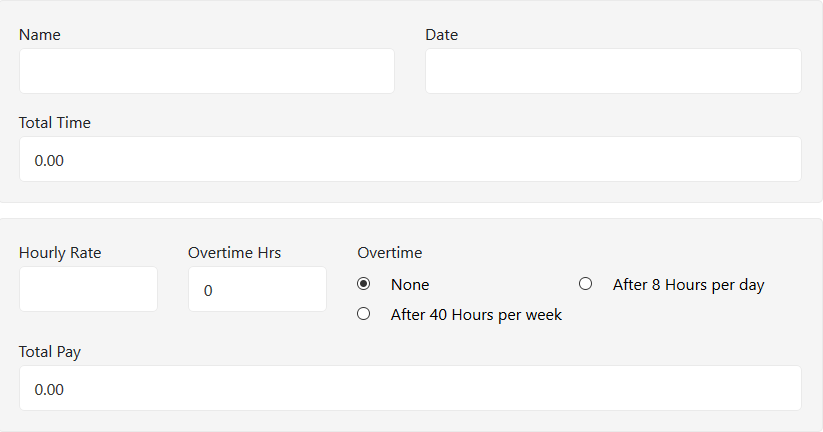5. Next, you need to select your Overtime calculation method, whether you are paid for every excess hour for 8 hours workday or 40 Hours per week.
6. Once selected, your overtime Hours will automatically be calculated and displayed under "Overtime Hrs" label.
7. Finally, enter your Hour Rate and determine your total pay, including overtime and paid lunch breaks.

Answer: According to Fair Labor Standards Act (FLSA), every excess hour worked over 8 hours in a day or 40 hours in a week, should be paid at time and half of the regular hourly rate as overtime to every Hourly Based Employee. Unfortunately, Salary based employees are exempted from this law.

Answer: Yes, it is absolutely illegal to not to pay overtime to eligible employees according to the Fair Labor Standards Act (FLSA). You can refer to their eligibility criteria for further details.

Answer: Working overtime can be good to complete pending work, earn extra bucks and give your employer a kind and hardworking impression for promotion or salary appraisal. However, it can be harmful. As it is wisely said, "Excess of everything is bad". Therefore, like everything, working excess overtime could severely affect your health and productivity. Moreover, you may not be paid for it if you are a salaried employee.

Answer: It can be any one of them. You may refer to your Employment contract, company’s policy or contact your HR.

Answer: There are several methods to calculate overtime. One of the methods is explained in FAQs Question # 2. Another two methods are a follow:

#### Method # 1: Calculating overtime hours after 8 hours per day

1. Record your Check-In and Check-Out time of work for a day on a piece of paper or spreadsheets, like Ms Excel.
2. Subtract your Check-In time from Check Out of work to get total hours worked in a day.

Suggestion: For quick subtraction, you can use our Time Duration Calculator.

1. If your regular hours worked according to your State law are 8 hours in a day, then the hours that exceed 8 hours in a day would be counted as overtime hours. Wherefore, subtract 8 hours from your total hours worked in a day to get overtime hours.
2. If you want to calculate your overtime pay, then convert your overtime hours into decimal overtime hours using our Time to Decimal Converter.
3. Finally, multiply your decimal overtime hours from 1 and half times of your regular hourly rate to deduce your overtime pay. For example:

If your decimal overtime hours are 3.5 Hours, and your regular hourly rate is \$20, then you need to:

Hourly Rate for overtime: 20 x 1.5 = \$30

Overtime Pay = 3.5 x 30 = \$105

#### Method # 2: Calculating overtime hours after 40 hours per week

1. Record your Check-In and Check-Out time of work for each day in a week on a piece of paper or spreadsheets, like Ms Excel.
2. Subtract your Check-In time from Check-Out time of work to get total hours worked in a day.

Suggestion: For quick subtraction, you can use our Time Elapsed Calculator.

1. Repeat Step 2 for each day in a week to get seven durations of hours worked in a day.
2. Now add together all durations of hours worked in a day to get Total Hours worked in a week.

Suggestion: you can use our hours calculator to get an instant and accurate result.

1. If your regular hours worked according to your State law are 40 hours in a week than the hours that exceed 40 hours In week would be counted as overtime hours. Wherefore, subtract 40 hours from your total hours worked in a week to get overtime hours.
2. If you want to calculate your overtime pay, then convert your overtime hours into decimal overtime hours using our Time to Decimal Converter.
3. Finally, multiply your decimal overtime hours from 1 and half times of your regular hourly rate to deduce your overtime pay. For example:

If your decimal overtime hours are 15.30 Hours, and your regular hourly rate is \$20, then you need to:

Hourly Rate for overtime: 20 x 1.5 = \$30

Overtime Pay = 15.3 x 30 = \$459

Answer: No, Taxes on overtime are the same as regular time for nonexempt employees (Hourly employees).

Answer: There are numerous methods to calculate overtime on Ms Excel. Here are two of them:

#### Method # 1: Calculating overtime hours after 8 hours per day on Ms Excel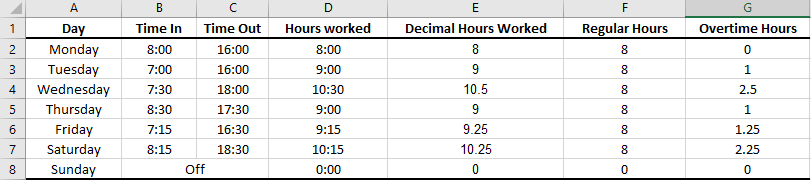We will be using the above image as an example. (You may alter it according to your need).

1. Make a Table, with column and row headings as shown in the image above.
2. Next record and input your Time In and Time Out of your work in 24 Hours format (Military Time) for your desired number of days. (In this example, we have recorded it for a week).
3. Calculate Hours Worked in a Day by subtracting Time In from their own Time Out by using the formula on Cell D2: =C2-B2. Use a drag and drop handle (a black dot on the bottom corner of the selected cell) to copy and paste the formula to the complete cell range from Cell D2 to D8.
4. Next, convert the Hours Worked durations into decimal hours by using the formula on Cell E2: =CONVERT(D2, "day", "hr"). Use the drag and drop handle again to copy and paste the formula to the complete cell range from Cell E2 to E8.
5. Next, input your regular hours, that is commonly 8 Hours a day in most states of the United States of America.
6. Finally, calculate your overtime hours for each day by inputting the formula: =(E2-F2) on Cell G2 and copy-pasting it on complete range from Cell G2 to G8, using the handle.

#### Method # 2: Calculating overtime hours after 40 hours per week on Ms Excel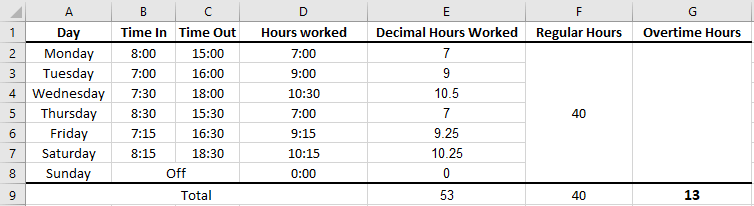We will be using the above image as an example. (You may alter it according to your need).

1. Make a Table, with column and row headings as shown in the image above.
2. Next record and input your Time In and Time Out of your work in 24 Hours format (Military Time) for each day in a week.
3. Calculate Hours Worked in a Day by subtracting Time In from their own Time Out by using the formula on Cell D2: =C2-B2. Use a drag and drop handle (a black dot on the bottom corner of the selected cell) to copy and paste the formula to the complete cell range from Cell D2 to D8.
4. Next, convert the Hours Worked durations into decimal hours by using the formula on Cell E2: =CONVERT(D2, "day", "hr"). Use the drag & drop handle again to copy and paste the formula to the complete cell range from Cell E2 to E8.
5. Next, select cell range from Cell F2 to F8 and press "Merge and Center" button under Alignment section of Home tab to merge complete range as one.
6. Now, input your regular hours, that is commonly 40 Hours a week in most states of the United States of America.
7. Now, calculate your total decimal Hours worked in a week by inputting the formula in Cell E9: =SUM(E2:E8). And re-enter or paste your regular hours in a week by inputting the formula in Cell F9: =F2.
8. Finally, calculate your overtime hours for a week by inputting the formula: =SUM(E9-F9) on Cell G9.

Answer: In bi-weekly pay, two weeks each having 40 hours as regular hours must be considered separately instead of combined 80 hours in bi-week (which is illegal). Therefore, If a non-exempted employee works 45 hours in the first week and 35 hours in the second week, he must be paid overtime for extra 5 hours for the first week.

Answer: According to the Fair Labor Standards Act (FLSA), employers are bound to pay overtime to non-exempted employees (including Hourly Based employees). However, there is no such law for salary-based employees. Therefore, unpaid overtime is legal for salary based (exempted employees) and illegal for non-exempted employees.

Answer: to calculate overtime on monthly pay period follow the steps below:

1. Record your Check-In and Check-Out time of work for each day in a month on a piece of paper or spreadsheet, like Ms Excel.
2. Subtract your Check-In time from Check-Out time of work to get total hours worked in a day.

Suggestion: For quick subtraction, you can use our Elapsed Time Duration Calculator.

1. Repeat Step 2 for each day in a month to get 29, 30 or 31 durations of hours worked in a day.
2. There are two overtime determination routines in the United States; one is to pay overtime for every hour that exceeds 8 hours of workday, and the other is to pay overtime for every hour that exceeds 40 hours of the workweek. However, for overtime on a monthly pay period, it is ideal to pay overtime for every hour that exceeds 8 hours of the workday as it covers each day of a month.
3. So, If your regular hours worked according to your State law are 8 hours in a day, then the hours that exceed 8 hours in a day would be counted as overtime hours. Wherefore, subtract 8 hours from your total hours worked in a day (for each day in the month) to get overtime hours (for each day In a month).
4. Now add together all values of overtime hours (for each day In a month) to get total overtime hours in a month.

Suggestion: you can use our hours calculator to get an instant and accurate result.

1. If you want to calculate your overtime pay than convert your total overtime hours in a month into decimal overtime hours in a month using our Time to Decimal Converter.
2. Finally, multiply your decimal overtime hours in a month from 1 and half times of your regular hourly rate to deduce your overtime pay for a month. For example:

If your decimal overtime hours for a month are 50.5 Hours, and your regular hourly rate is \$20, then you need to:

Hourly Rate for overtime: 20 x 1.5 = \$30

Overtime Pay = 50.5 x 30 = \$1515

Answer: There is no such law for overtime in bi-monthly pay period Fair Labor Standards Act (FLSA). Therefore, companies follow 40 hours in week law, where an employer pays for every exceeding hours after 40 hours worked in a week to their non-exempted (hourly based) employees.

However, there is one problem, in this bi-monthly payment method, that may jeopardize the employer to break the law. In this method, the employer pays, every 15th and 1st date of the month, so they pay for 15 days and count overtime for 14 days (2 weeks), which is against the law and incurs a loss to the employee. Therefore, employers are required to use a computerized system, that considers overtime for each day and pays it accordingly.

Other Calcutors